# Determination of quantity supplied by firm in perfectly competitive market in the short run with marginal cost that decreases then increases

This is the development of a special case of determination of quantity supplied by firm in perfectly competitive market in the short run. The notation builds on notation used in that page.

## Recall of assumptions and setup

We do not recall all the definitions, but we do recall the key assumptions:

• The firm can choose to produce any quantity between$0$ and a maximum quantity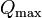$Q_{\max}$.
• The marginal cost$MC$ is defined at all quantities of production in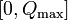$[0,Q_{\max}]$. Formally, it is the derivative of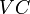$VC$ with respect to$Q$, though at the endpoints$0$ and$Q_{\max}$, we take one-sided derivatives.
• For simplicity, we also assume that the$MC$ curve is continuous and is not highly oscillatory, i.e., we can break up the interval$[0,Q_\max]$ into finitely many pieces and the curve is either increasing or decreasing on each piece.

## Key general principle for profit maximization

The absolute (i.e., global) maximum for profit must occur either at one of these three points: zero quantity of production, the maximum quantity of production, or some local maximum at an intermediate quantity of production. In the last case, that must be a point where$P = MC$ and the$MC$ curve is rising.

### Description of case

Suppose that, for low quantities of production, the marginal cost decreases, i.e., the marginal cost initially falls, and then, for larger quantity of production, the marginal cost increases. In this case, the picture has the following features:

• Both the marginal cost and the average variable cost start out from the same point (on the vertical price axis).
• Initially, the marginal cost curve and average variable cost are both downward-sloping, with the marginal cost curve going down faster than the average variable cost curve.
• At some point, the marginal cost curve turns around and starts moving upward. At this stage, the average variable cost curve is still downward-sloping because the marginal cost is still lower than the average variable cost.
• At a slightly later stage, the marginal cost becomes equal to the average variable cost, and this is the same as the point at which the average variable cost starts increasing. The value of marginal cost and average variable cost at this stage turns out to be the reservation price. The corresponding quantity of production is termed the minimum efficient scale. For an explanation of why the MC and AVC curves must cross precisely at the point of minimum AVC, see comparison of marginal and average determines direction of change of average.
• Beyond this, both the marginal cost and the average variable cost are increasing, with the marginal cost increasing faster than the average variable cost.

## Profit-maximizing choice

### Summary of choices

In the table below, cases (1) and (2) are not qualitatively different as far as the final outcome is concerned, but the formal mathematical analysis differs somewhat. Cases (3) and (4) are not qualitatively different as far as the final outcome is concerned, but the formal mathematical analysis differs somewhat.

Case no. Case for relation between market price and marginal cost curve Quantity of production chosen to maximize profits Explanation in terms of formal mathematical analysis
1 The market price is less than the minimum value of MC Zero. In other words, the firm stays idle and waits for the market price to rise. The profit function is decreasing on the entire interval$[0,Q_\max]$, because its derivative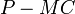$P - MC$ is negative throughout, so clearly the local and global profit-maximizing choice is to produce nothing. So, this case is quite clear.
2 The market price is greater than the minimum value of MC but smaller than the minimum value of AVC. Zero. In other words, the firm stays idle and waits for the market price to rise. The behavior of the profit function is as follows: decreasing from 0 to the quantity at which$P = MC$ ($MC$ falling), increasing from that quantity to the quantity at which$P = MC$ ($MC$ rising), and decreasing again from that to$Q_\max$. Thus, the only candidates for the maximum are 0 and the point where$P = MC$,$MC$ rising. The profit function (not accounting for fixed costs) is 0 at 0 and is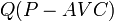$Q(P - AVC)$ at the point where$P = MC$ ($MC$ rising). The latter is negative, hence smaller than the value at zero, so the profit-maximizing choice is to produce nothing.
3 The market price is greater than the minimum value of AVC but less than the value of MC for quantity zero. Determine the quantity at which the marginal cost equals the market price. Pick the quantity value that is to the right of the minimum efficient scale, i.e., the quantity at which minimum AVC is attained (See picture) The behavior of the profit function is as follows: decreasing from 0 to the quantity at which$P = MC$ ($MC$ falling), increasing from that quantity to the quantity at which$P = MC$ ($MC$ rising), and decreasing again from that to$Q_\max$. Thus, the only candidates for the maximum are 0 and the point where$P = MC$,$MC$ rising. The profit function (not accounting for fixed costs) is 0 at 0 and is$Q(P - AVC)$ at the point where$P = MC$ ($MC$ rising). The latter is positive, hence bigger, hence that is the optimizing point.
4 The market price is greater than the minimum value of AVC and greater than the value of MC for quantity zero but less than the value of MC for maximum quantity produced. Determine the unique quantity at which the marginal cost equals the market price. The behavior of the profit function is as follows: increasing from 0 to the quantity at which$P = MC$ ($MC$ rising), then decreasing till$Q_\max$. Thus, the quantity at which$P = MC$ is the unique profit-maximizing quantity.
5 Even at the maximum quantity that the firm can produce, the market price is more than the marginal cost. Produce at the maximum quantity. The profit function is increasing on the interval$[0,Q_\max]$, since the derivative$P - MC$ is positive throughout. Hence, it is maximum at$Q_\max$.

### Key price and quantity values

• The reservation price of the firm is the minimum value of AVC.
• The minimum efficient scale, defined as the smallest nonzero quantity the firm produces in a profit-maximizing choice, is the quantity value corresponding to minimum AVC.

### Nature of short run supply curve

The short run supply curve is along the price axis for price less than minimum AVC, then at minimum AVC it jumps to the minimum AVC point, and thereafter, it travels along the marginal cost curve. At price levels higher than the marginal cost for$Q_\max$, it is vertical with quantity coordinate$Q_\max$.